# Basic Logic Gate - AND OR NOT

Logic Gate

In this tutorial we will learn about the basic logic gates - AND OR NOT

## Logic Gate: AND

The logic gate AND takes two or more input and works as per the following truth table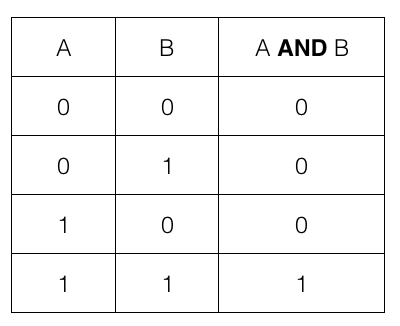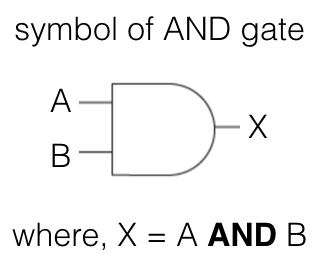i.e., if both the input A and B are 1 or TRUE then the output is 1 or TRUE; otherwise it is 0 or FALSE

where, 0 means OFF or FALSE and 1 means ON or TRUE

We can visualise the two input of AND gate as two switches connected in series. If both the switches are turned ON (i.e., TRUE or 1) then only the bulb will glow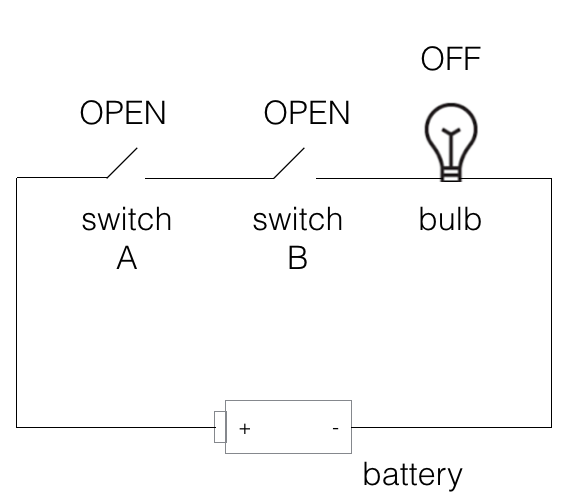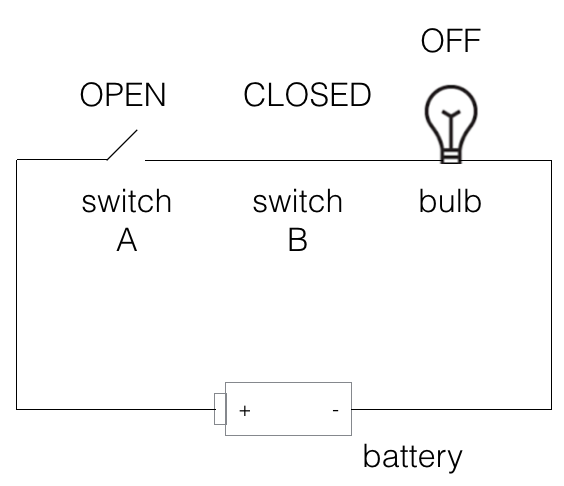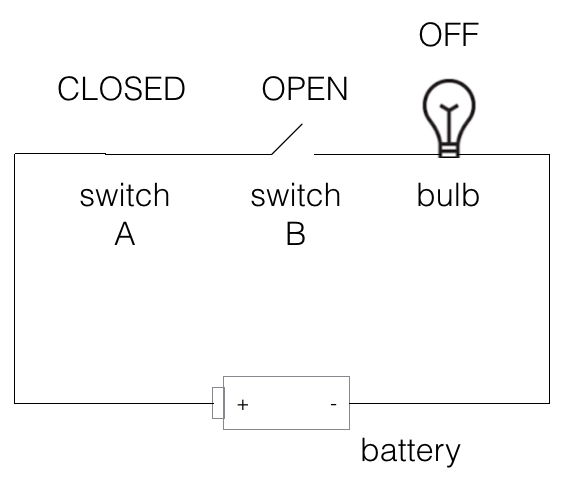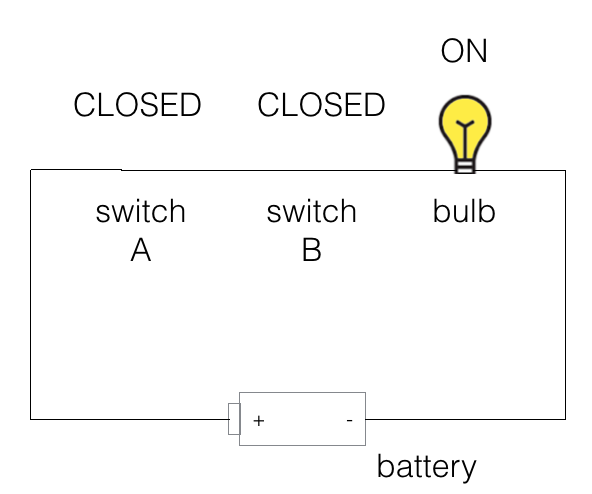## AND in code

We denote AND using && in programming language like C, C++, Java, JavaScript, Php etc.

So, if we are given an if-statement then the code inside the if block will be executed if both the variables (expression) are true.

``````
//javascript
var A = true;
var B = true;
if(A && B) {
console.log("Hello");
} else {
console.log("Bye");
}
``````

The given code will print Hello as both A and B are true.

## Logic Gate: OR

The logic gate OR takes two or more input and works as per the following truth table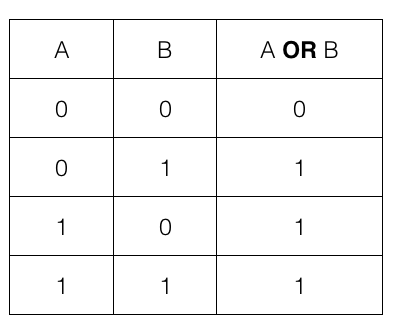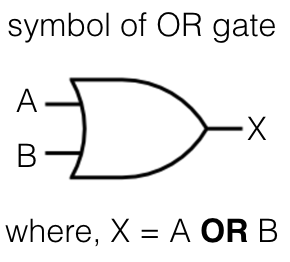i.e., if any one of the input A or B is 1 or TRUE then the output is 1 or TRUE; otherwise it is 0 or FALSE

We can visualise the two input of OR gate as two switches connected in parallel. If any one of the switches is turned ON (i.e., TRUE or 1) then the bulb will glow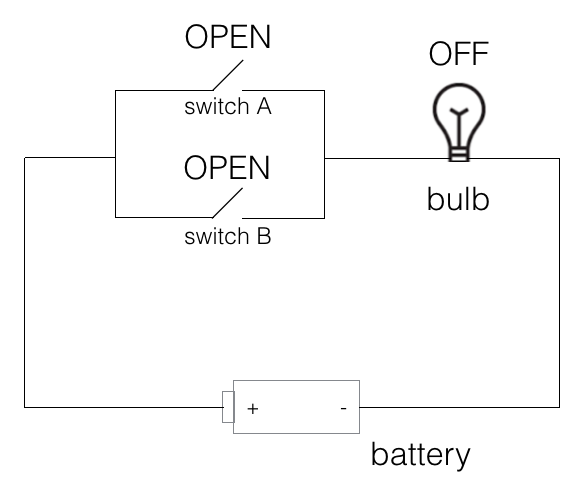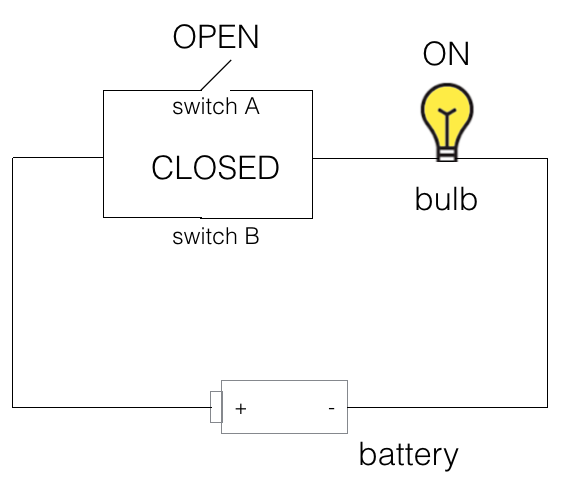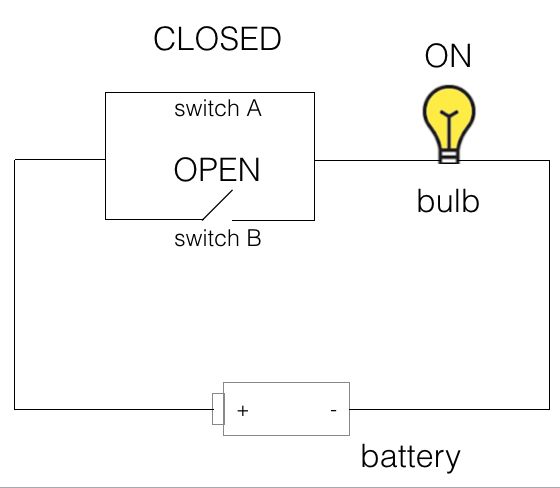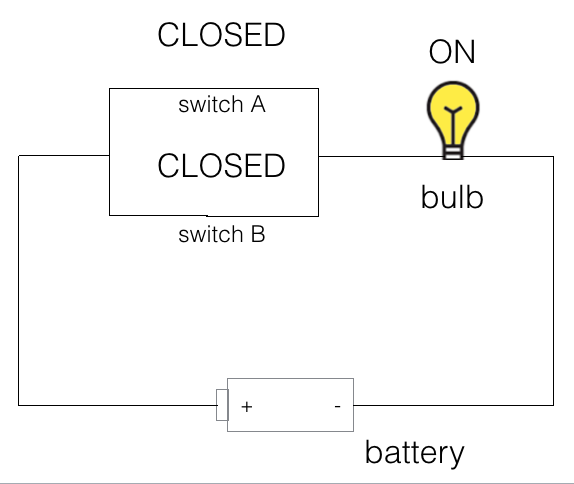## OR in code

we denote OR using || in programming language like C, C++, Java, JavaScript, Php etc.

So, if we are given an if-statement then the code inside the if block will be executed if any one of the variable (expression) is true.

``````
//javascript
var A = true;
var B = false;
if(A || B) {
console.log("Hello");
} else {
console.log("Bye");
}
``````

The given code will print Hello as A is true.

## Logic Gate: NOT

The logic gate NOT takes only one input and works as per the following truth table.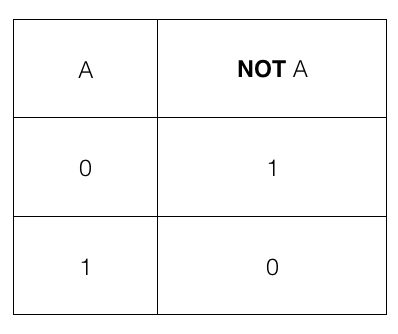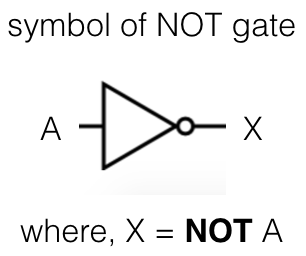i.e., if the input A is 1 or TRUE then the output is 0 or FALSE and if the input A is 0 or FALSE then the output is 1 or TRUE

## NOT in code

we denote NOT using ! in programming language like C, C++, Java, JavaScript, Php etc.

So, if we are given an if-statement then the code inside the if block will be executed if the variable (expression) is false.

``````
//javascript
var A = false;
if(!A) {
console.log("Hello");
} else {
console.log("Bye");
}
``````

The given code will print Hello as A is false because NOT of false is true.